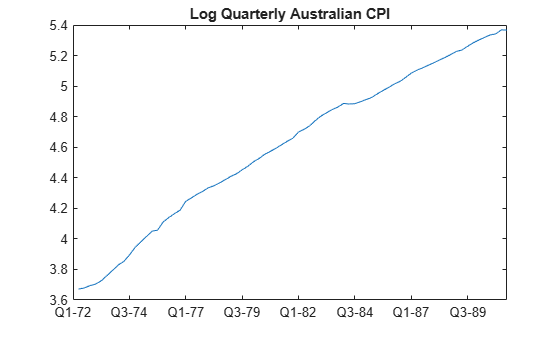# Box-Jenkins Differencing vs. ARIMA Estimation

This example shows how to estimate an ARIMA model with nonseasonal integration using `estimate`. The series is not differenced before estimation. The results are compared to a Box-Jenkins modeling strategy, where the data are first differenced, and then modeled as a stationary ARMA model (Box et al., 1994).

The time series is the log quarterly Australian Consumer Price Index (CPI) measured from 1972 through 1991.

Load and plot the Australian CPI data.

```load Data_JAustralian y = DataTable.PAU; T = length(y); figure plot(y); h = gca; % Define a handle for the current axes h.XLim = [0,T]; % Set x-axis limits h.XTickLabel = datestr(dates(1:10:T),17); % Label x-axis tick marks title('Log Quarterly Australian CPI')```The series is nonstationary, with a clear upward trend. This suggests differencing the data before using a stationary model (as suggested by the Box-Jenkins methodology), or fitting a nonstationary ARIMA model directly.

### Estimate an ARIMA Model

Specify an ARIMA(2,1,0) model, and estimate.

```Mdl = arima(2,1,0); EstMdl = estimate(Mdl,y);```
``` ARIMA(2,1,0) Model (Gaussian Distribution): Value StandardError TStatistic PValue __________ _____________ __________ _________ Constant 0.010072 0.0032802 3.0707 0.0021356 AR{1} 0.21206 0.095428 2.2222 0.026271 AR{2} 0.33728 0.10378 3.2499 0.0011543 Variance 9.2302e-05 1.1112e-05 8.3066 9.849e-17 ```

The estimated model is

`$\Delta {y}_{t}=0.01+0.21\Delta {y}_{t-1}+0.34\Delta {y}_{t-2}+{\epsilon }_{t},$`

where ${\epsilon }_{t}$ is normally distributed with standard deviation 0.01.

The signs of the estimated AR coefficients correspond to the AR coefficients on the right side of the model equation. In lag operator polynomial notation, the fitted model is

`$\left(1-0.21L-0.34{L}^{2}\right)\left(1-L\right){y}_{t}={\epsilon }_{t},$`

with the opposite sign on the AR coefficients.

### Difference the Data Before Estimating

Take the first difference of the data. Estimate an AR(2) model using the differenced data.

```dY = diff(y); MdlAR = arima(2,0,0); EstMdlAR = estimate(MdlAR,dY);```
``` ARIMA(2,0,0) Model (Gaussian Distribution): Value StandardError TStatistic PValue __________ _____________ __________ _________ Constant 0.010429 0.0038043 2.7414 0.0061183 AR{1} 0.20119 0.10146 1.9829 0.047375 AR{2} 0.32299 0.11803 2.7364 0.0062115 Variance 9.4242e-05 1.1626e-05 8.1062 5.222e-16 ```

The parameter point estimates are very similar to those in `EstMdl`. The standard errors, however, are larger when the data is differenced before estimation.

Forecasts made using the estimated AR model (`EstMdlAR`) will be on the differenced scale. Forecasts made using the estimated ARIMA model (`EstMdl`) will be on the same scale as the original data.

References:

Box, G. E. P., G. M. Jenkins, and G. C. Reinsel. Time Series Analysis: Forecasting and Control. 3rd ed. Englewood Cliffs, NJ: Prentice Hall, 1994.